# The Excel Skinny

A blog about Excel and its users

April 30, 2017

## VLOOKUP on Mac Excel

The VLOOKUP function, when mastered, is one of the most useful functions in Microsoft Excel.  A VLOOKUP is a function that works off the first column in a list of data.  When would you use a VLOOKUP?  When you are trying to pull specific data from a list into another cell.  For our example we will use an Invoice List: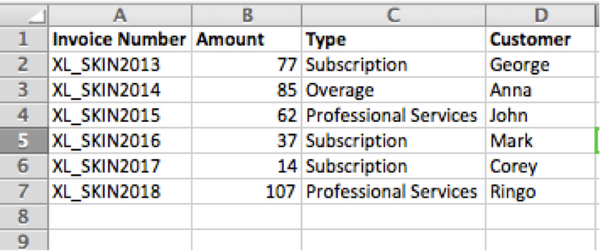For a VLOOKUP to work you must have a unique identifier and that unique identifier must be in the first column of your list.  In this example our unique identifier is the Invoice Number.  Once the VLOOKUP is executed if we put XL_SKIN2013 in a cell with the function it will return to us all the information we want.  Please note the VLOOKUP function has no restrictions whether you want to pull information into the same spreadsheet, same workbook, or different workbooks.

To start, we put our Unique Identifier into a new cell.  This will be the new list where we want to pull this information into.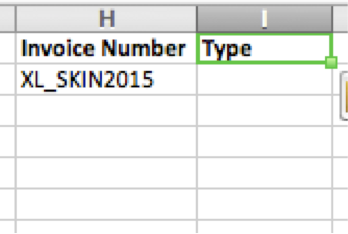The cell next to XL_SKIN2015 will be where we enter the VLOOKUP formula.  Refer back to the Formula Builder Shortcut to open the Formula Builder.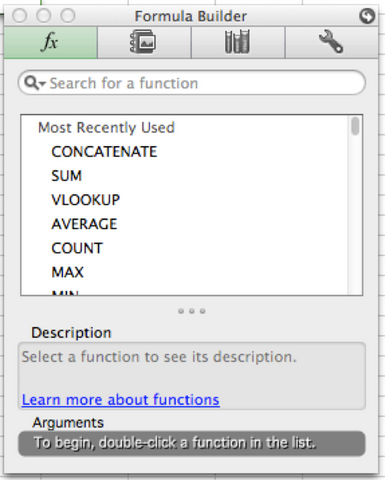Type VLOOKUP in the Search for a Function and double click VLOOKUP to start the function: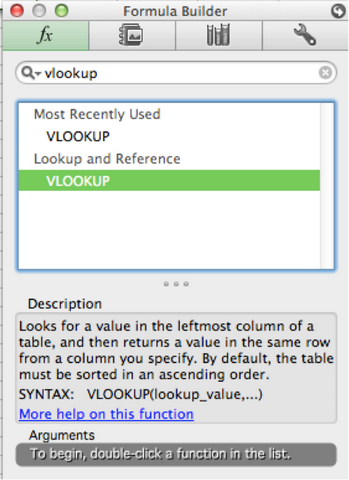The formula builder will ask you for lookup_value, table_array, col_index_num, and range_lookup(optional).  For these values insert:

• Lookup_value = the value to be found in the first column of the table, and can be a value, a reference, or a text string.
• If you have you click the white box next to the lookup_value you can then go to your new list (where you will be pulling information into) and select the Unique Identifier you have already inserted:• Table_array = Tell the VLOOKUP where the original database/list is.  Select the entire list for this. Do not include headers.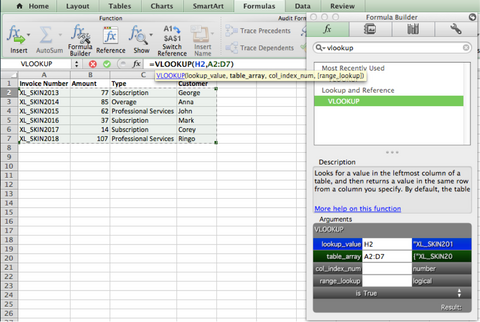• Col_index_num = this part of the VLOOKUP is to specify which information from the original database or list we want.  In our second list we said we want the Type of invoice, so we put 3 for Column 3.  Please note the col_index_num is always the number of columns in from your database or list.  It does not matter where the list starts or ends in relation to the Columns and Rows laid out from Microsoft Excel.
• Range_Lookup = This is either True or False.  Use the following parameters to decide if you need a value in the Range_Lookup:
• If the first column of the database (the column that contains the unique identifiers) is sorted alphabetically/numerically in ascending order, then it’s possible to enter a value of true into this argument, or leave it blank.
• If the first column of the database is not sorted, or it’s sorted in descending order, then you must enter a value of false into this argument
• Our first column in the database is sorted so we do not need a value here.

Press Enter and the information should appear in the VLOOKUP cell!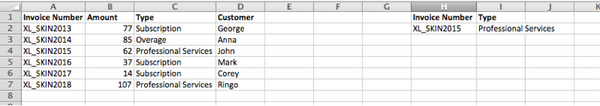If you would like to add more information to the new list simply drag the VLOOKUP down and keep adding Invoice Numbers.  The information will automatically populate: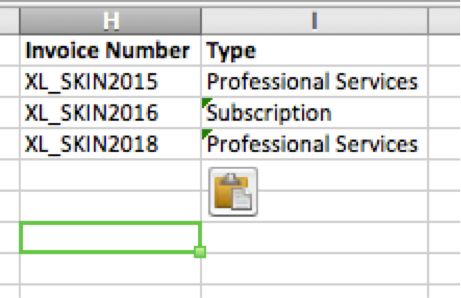## Using Logical Formulas in Microsoft Excel

September 28, 2016

## How do you compare data in Microsoft Excel? Logical Formulas!

Excel uses logical operations to ease the pain in comparing data. These formulas will return what we tell them to, based on specific criteria.

We will teach you how to run less than, greater than, and equal to formulas below.
For the first formula, we will focus on the “Greater Than” formula. This is used when you want to isolate cells that contain values greater than the parameters in your formula.
For the example below, I have a series of numbers between 1 and 10. All I want to know is if the number is greater than, or less than 2.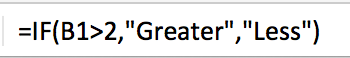As shown above, my formula is: =IF(B1>2,"Greater","Less") for the first cell. This is telling Excel, if the data in B1 is greater than 2, input the word “Greater”. If it is less than 2, input “Less”.  I then pulled this formula down by the square in the lower right hand side of the D1 cell to fit my list.

This is a very simple way to use the =IF logical formulas, but they can become extremely complex if needed.

As always, please let the Excel Skin team know if you have any questions!

## Toggle between cell values and formulas

September 15, 2013

This is a cool shortcut that can come in handy for any Excel users that have multiple formulas in a spreadsheet.  It’s very simple - all it does is toggles your whole spreadsheet so show either the cell values, or the cell formulas.  This effects the entire spreadsheet so any formula will be toggled back and forth when executing the shortcut.

This shortcut can be executed from anywhere on the spreadsheet.  To execute simply press:⌃`

Once executed your spreadsheet will change from values to formulas, or vice versa.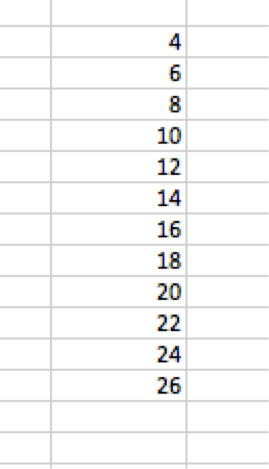toNow go have fun toggling back and forth between your formulas and values!

## Selecting cells related to a formula in the active cell

February 16, 2013

## How to highlight the cells related to a selected formula

This shortcut provides a very useful tool for those working with a plethora of formulas in their spreadsheets.  It will highlight all the cells that are involved in the formula of the active cell you have selected.  In the following steps the shortcut's effectivness will be more transparent.

Step 1: For this shortcut you must have a working formula in your spreadsheet.  I will demonstrate with the =SUM formula.

Step 2: Select the cell that contains the formula.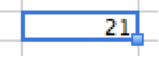To double-check there is a formula present in the cell, glance up at the function box. For this example my function box reads:Step 3: Now that I have selected a cell with a formula in it I can execute the “Referenced” shortcut to see all cells that correspond with this formula.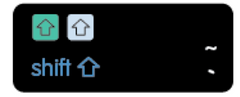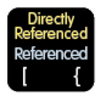⌃⇧{

Result: All the cells that are involved in my =SUM formula are now highlighted in the spreadsheet.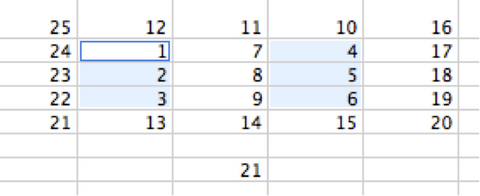This shortcut is especially useful in large seas of data when it may be hard to pinpoint all the cells related to a specific formula.